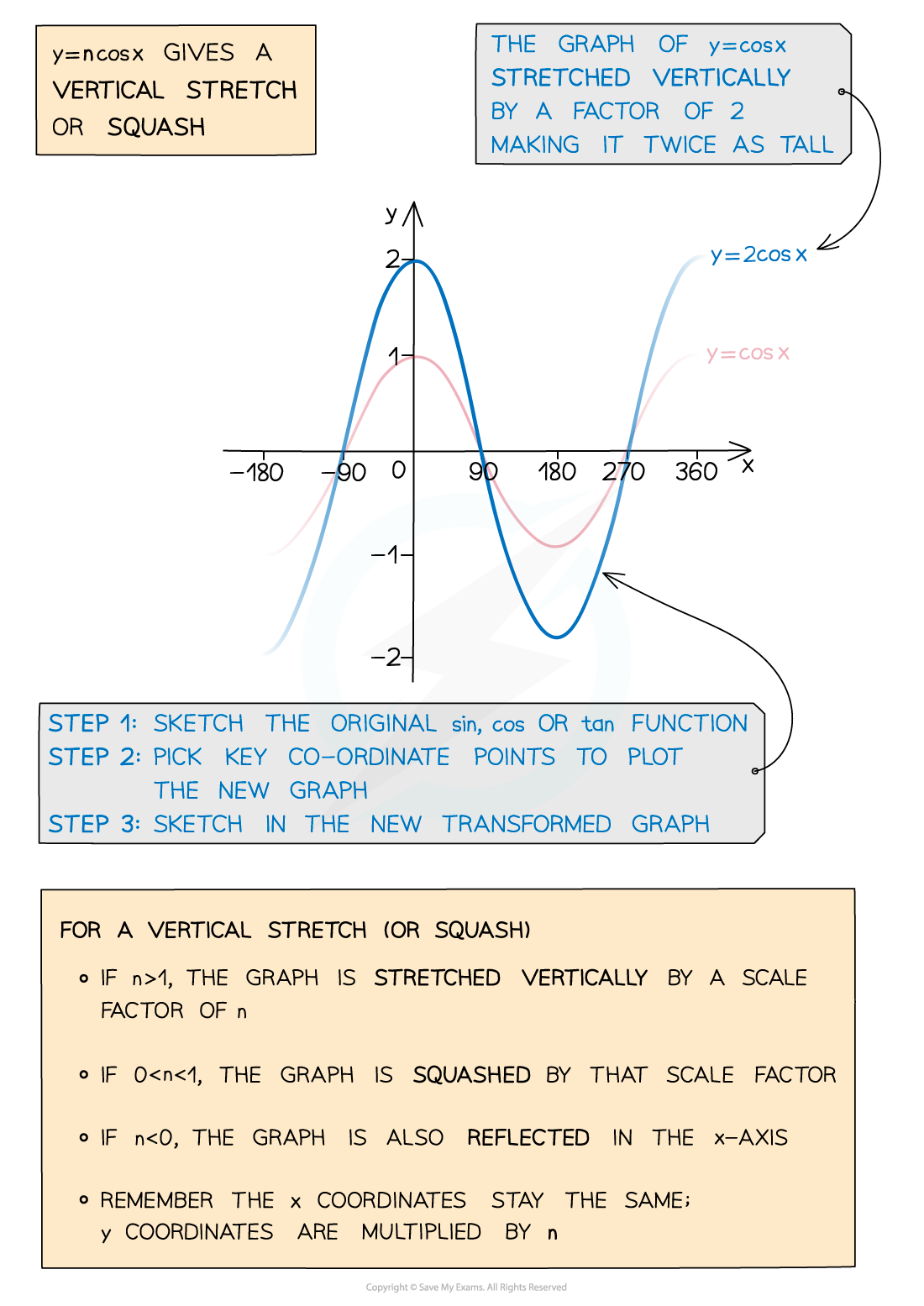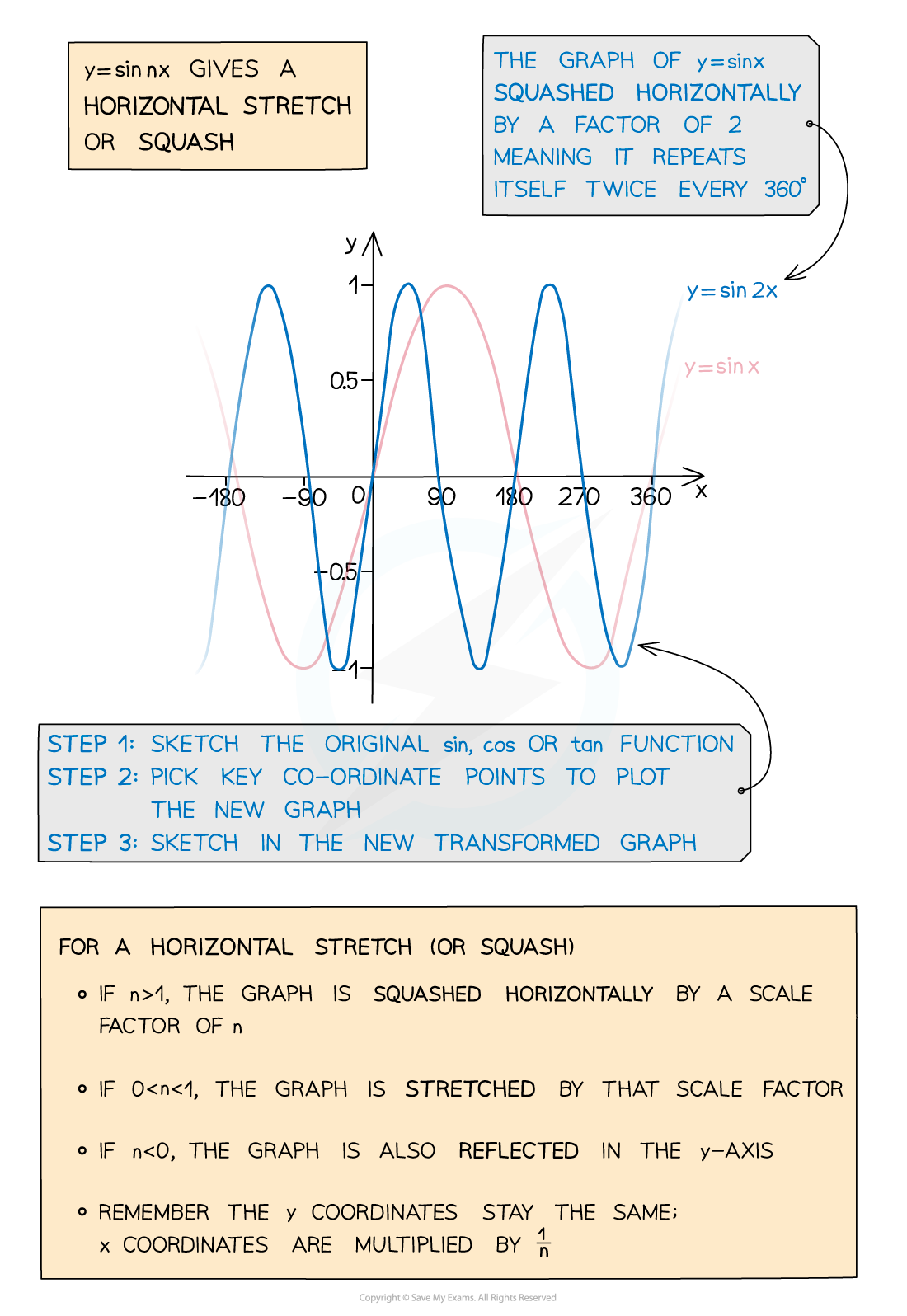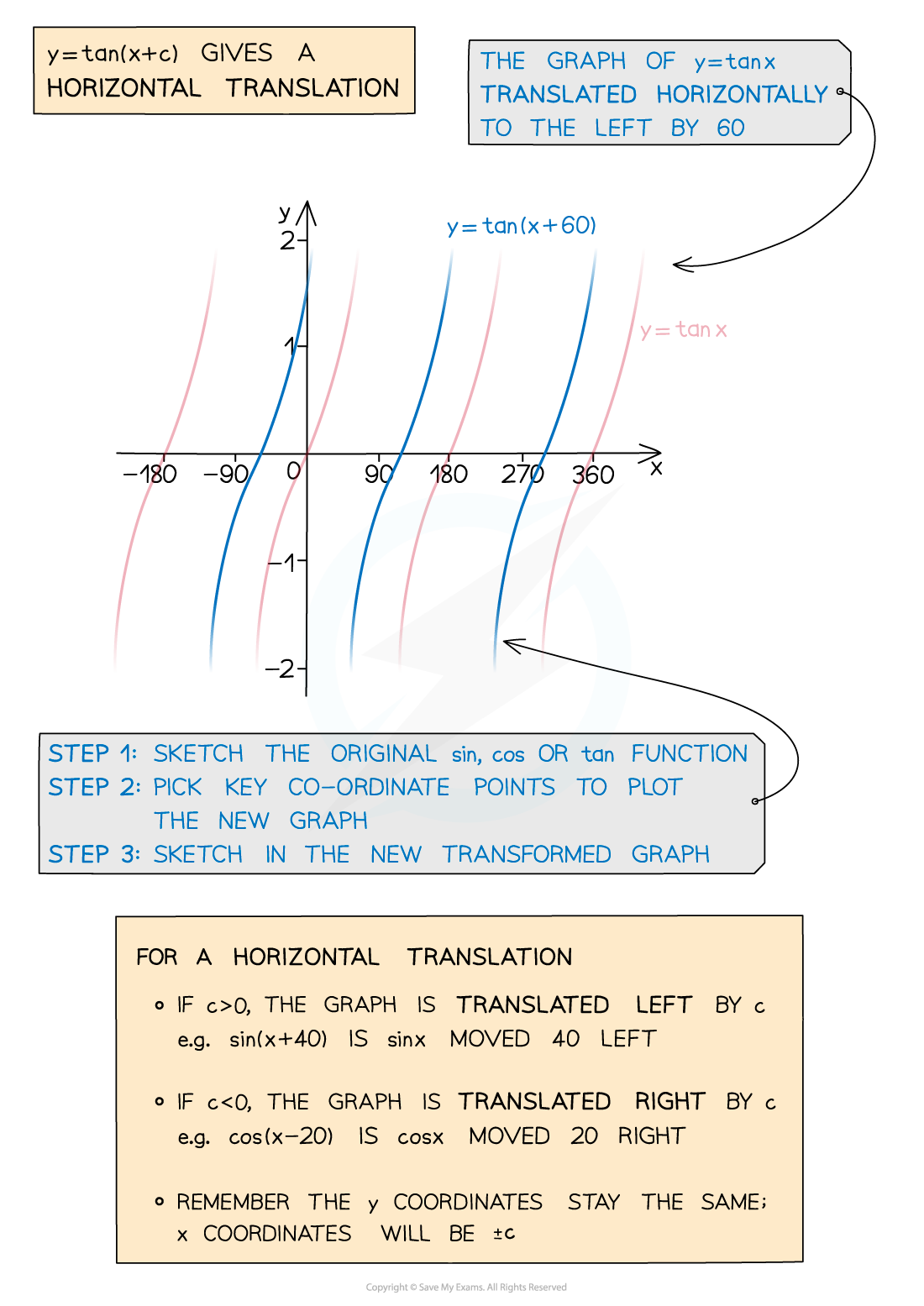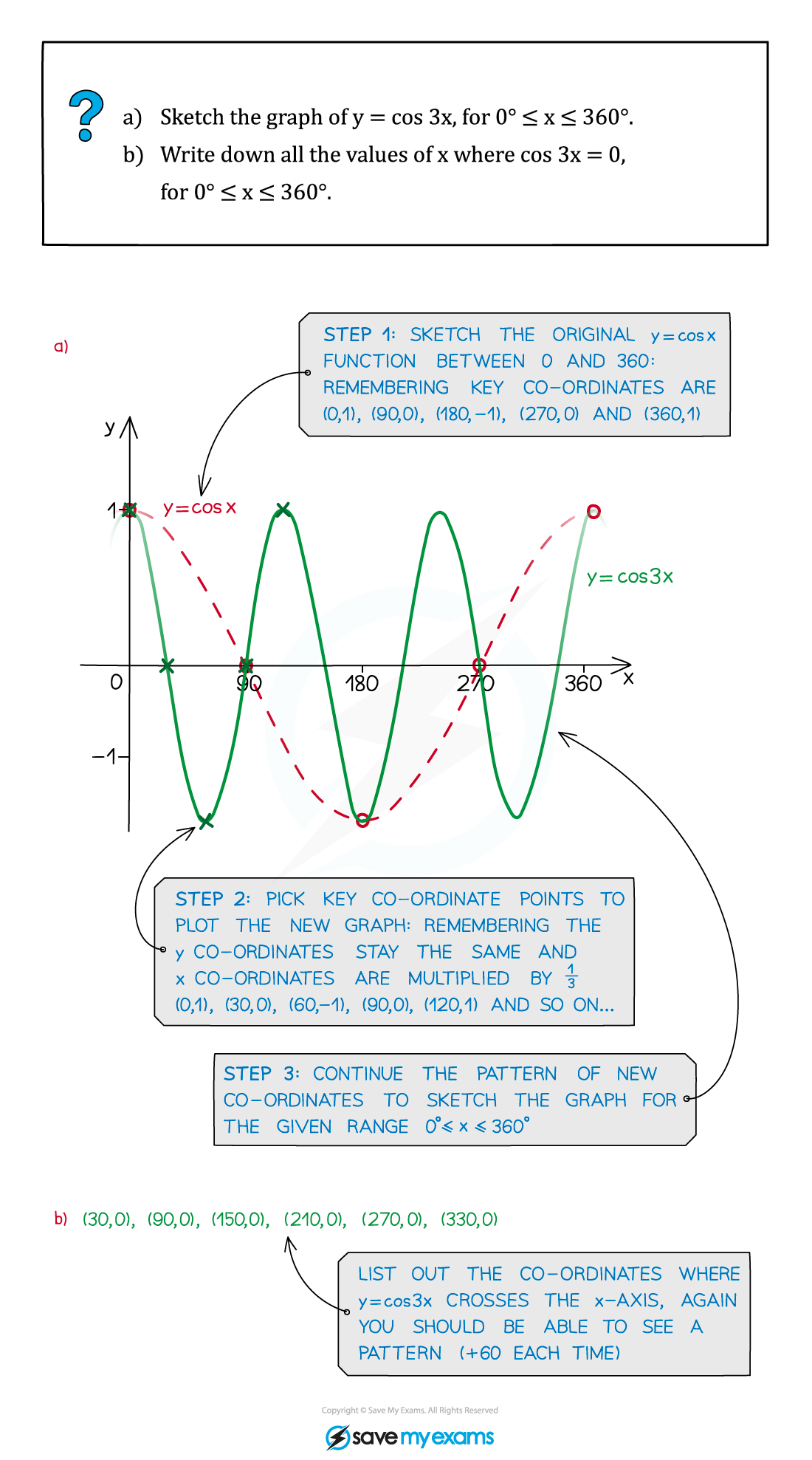# AQA A Level Maths: Pure复习笔记5.2.2 Transformations of Trigonometric Functions

### Transformations of Trigonometric Functions

#### Transformations of trigonometric functions

• You should already have a good idea about translating, stretching and reflecting basic functions (see Transformations of Functions)
• The basic principles are exactly the same for transforming any trigonometric graph
• y = n cos x gives a vertical stretch or squash
• y = sin nx gives a horizonal stretch or squash
• y = tan (x + c) gives a horizonal translation#### Exam Tip

• Always sketch with a pencil and draw a smooth curve.
• When you sketch the transformation of a graph, be sure to indicate the new coordinates of any points that are marked on the original graph.
• Try to indicate the coordinates of points where the new graph intersects the axes.

#### Worked Example